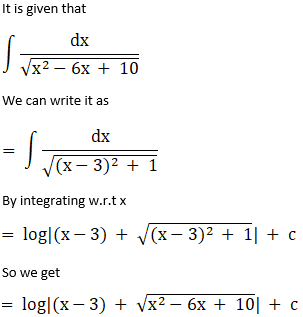# RS Aggarwal Solutions for Class 12 Maths Chapter 14: Some Special Integrals Exercise 14B

Mathematics will usually be fun for some students and a nightmare for others. The main reason for this is lack of understanding during class hours. To overcome this issue, students can download the solutions PDF designed by faculty at BYJU’S, while solving textbook problems. It mainly improves logical approach among students in answering problems easily. This exercise covers the three more special integrals as per CBSE guidelines. Students can download RS Aggarwal Solutions Class 12 Maths Chapter 14 Some Special Integrals Exercise 14B PDF, from the links which are available below.

## RS Aggarwal Solutions for Class 12 Maths Chapter 14: Some Special Integrals Exercise 14B Download PDF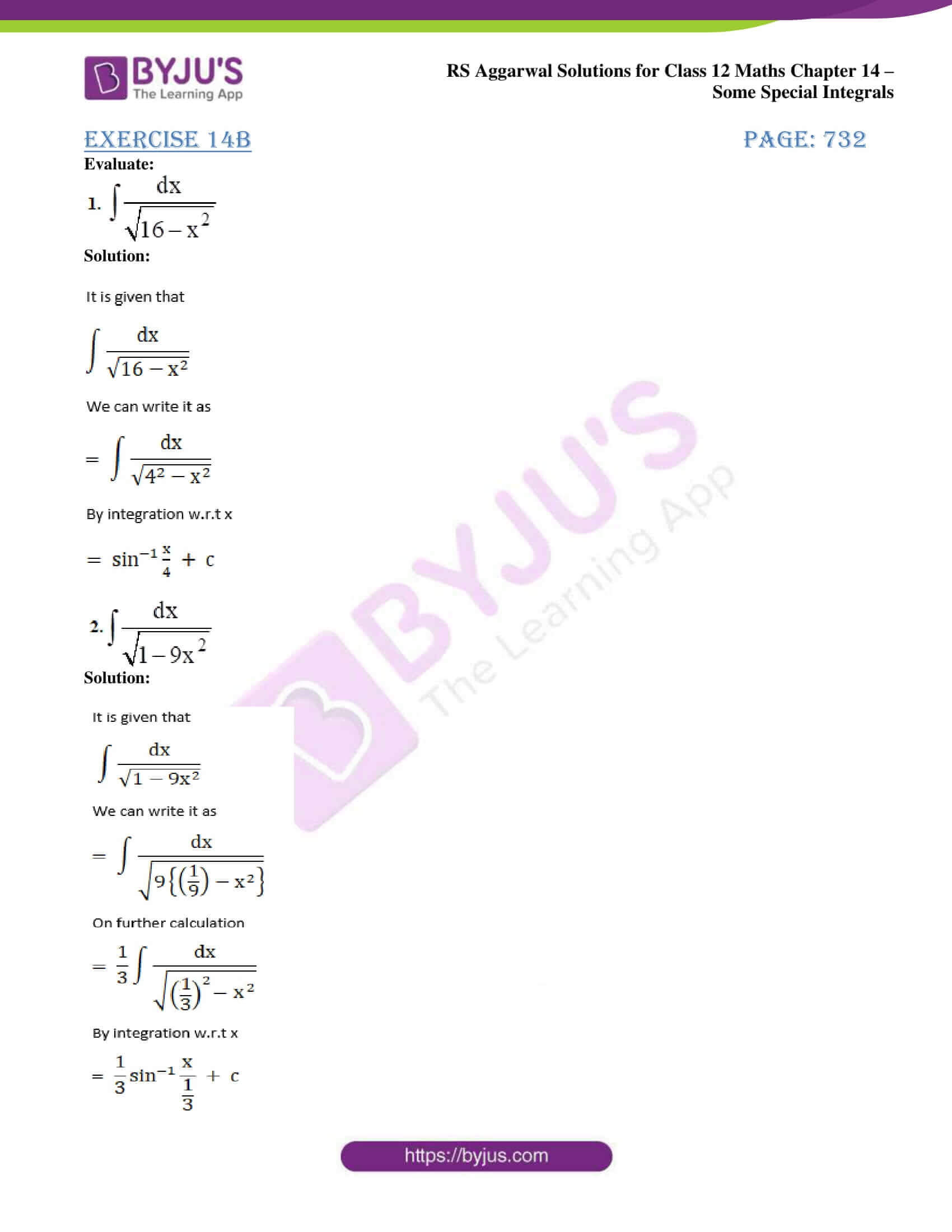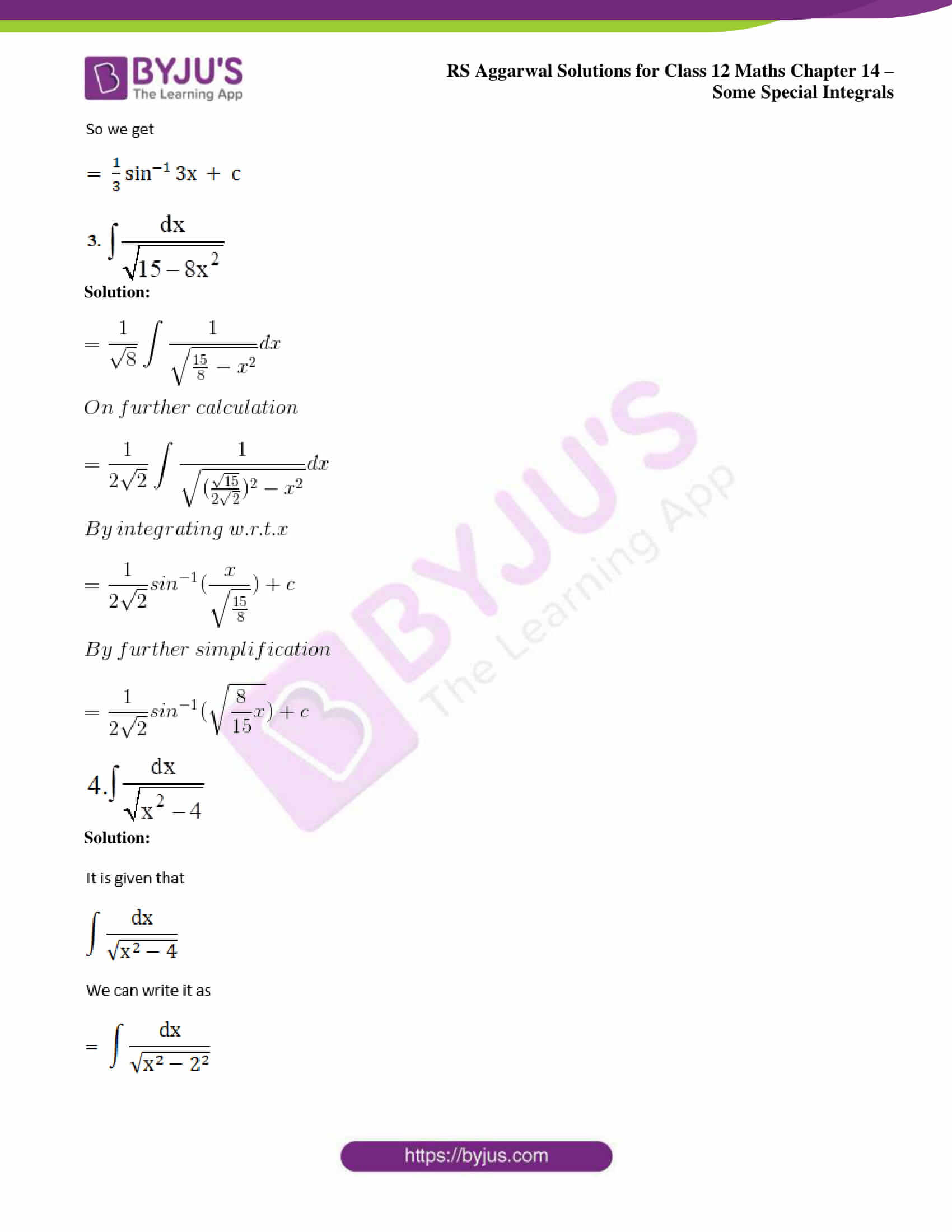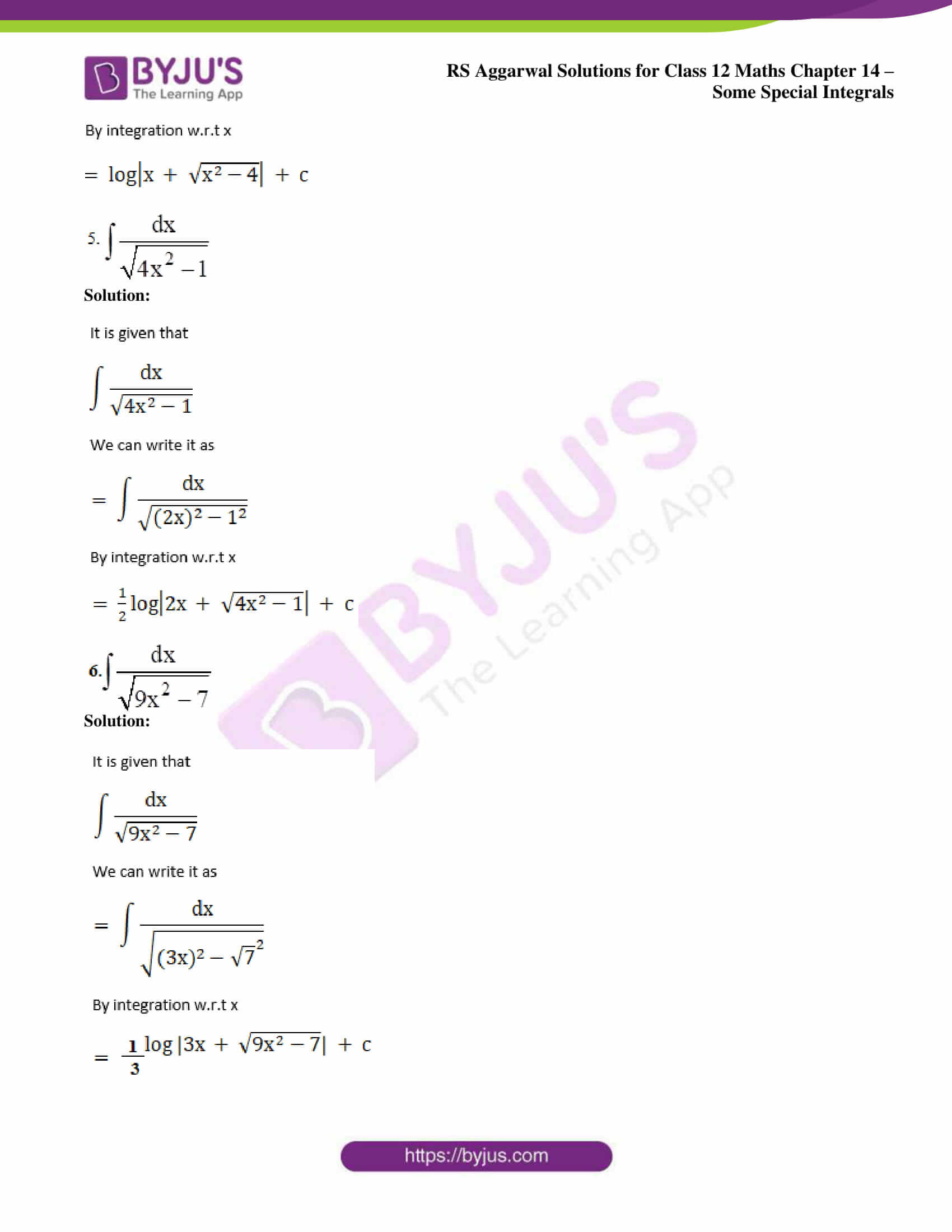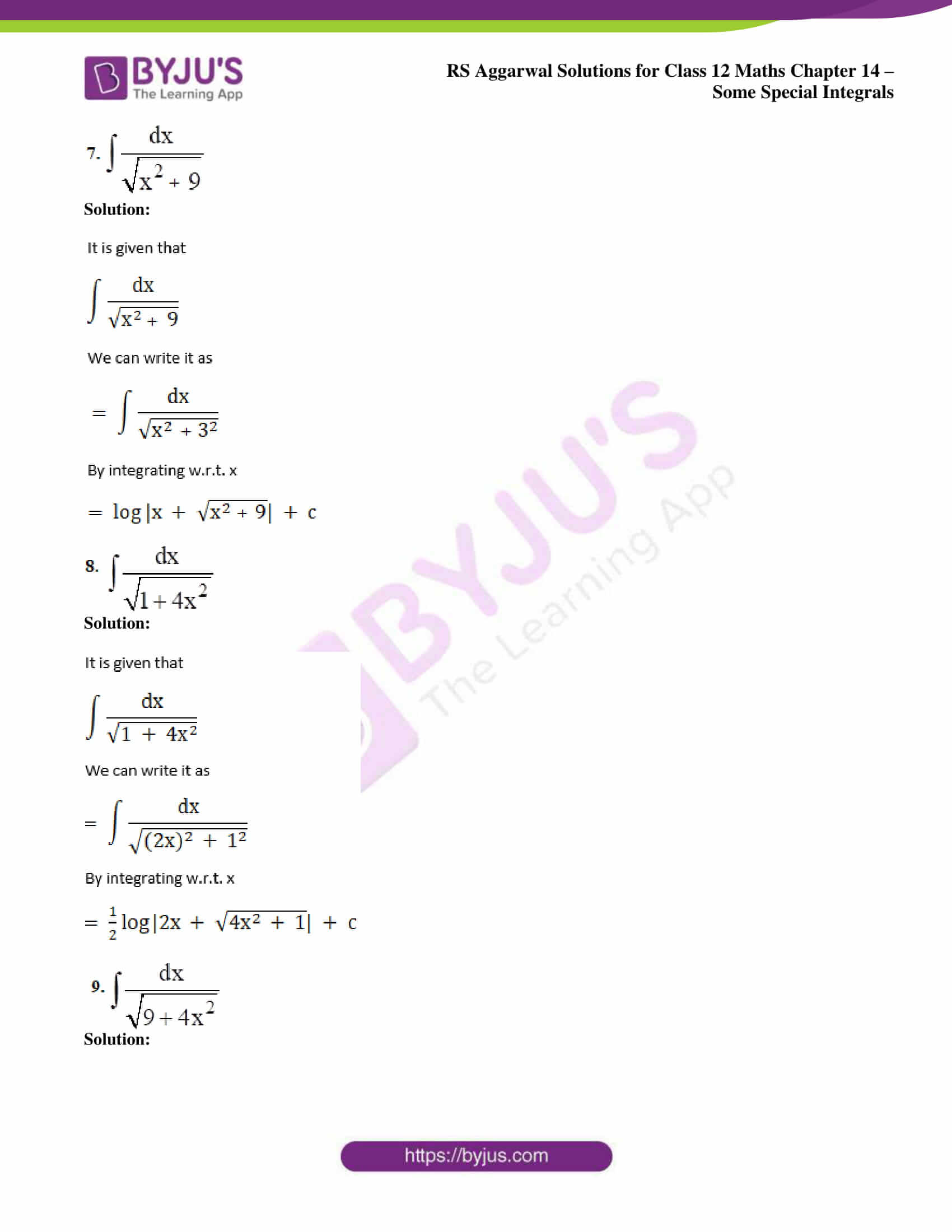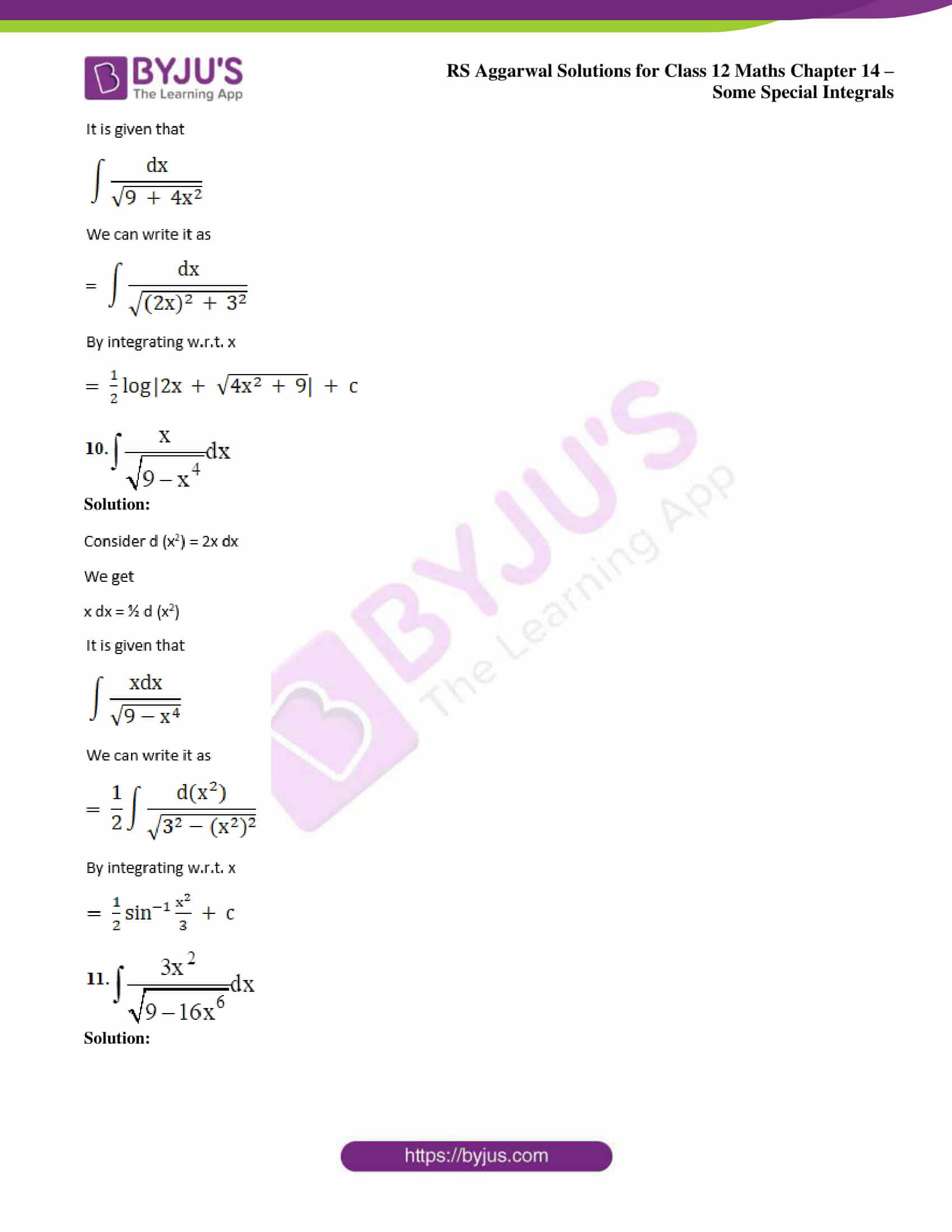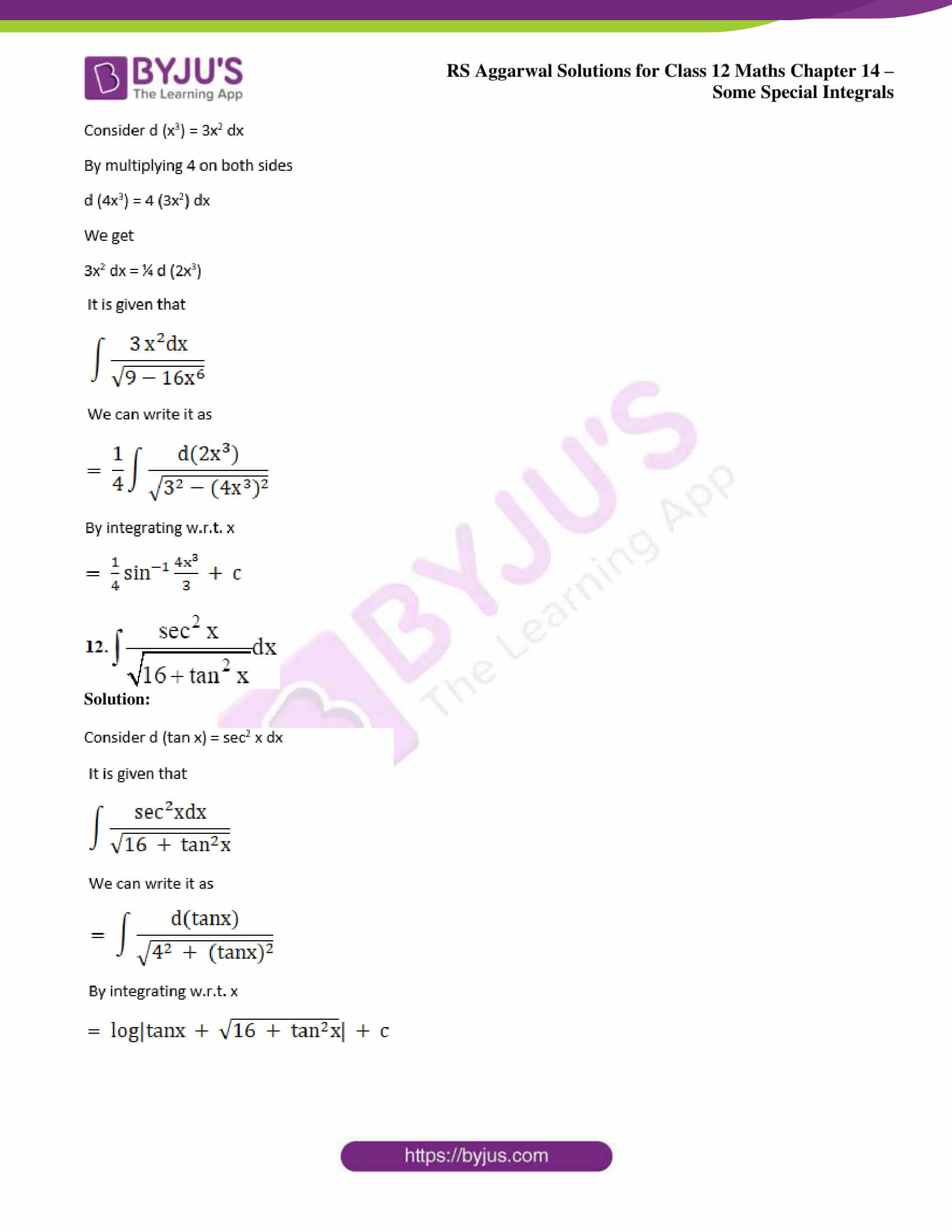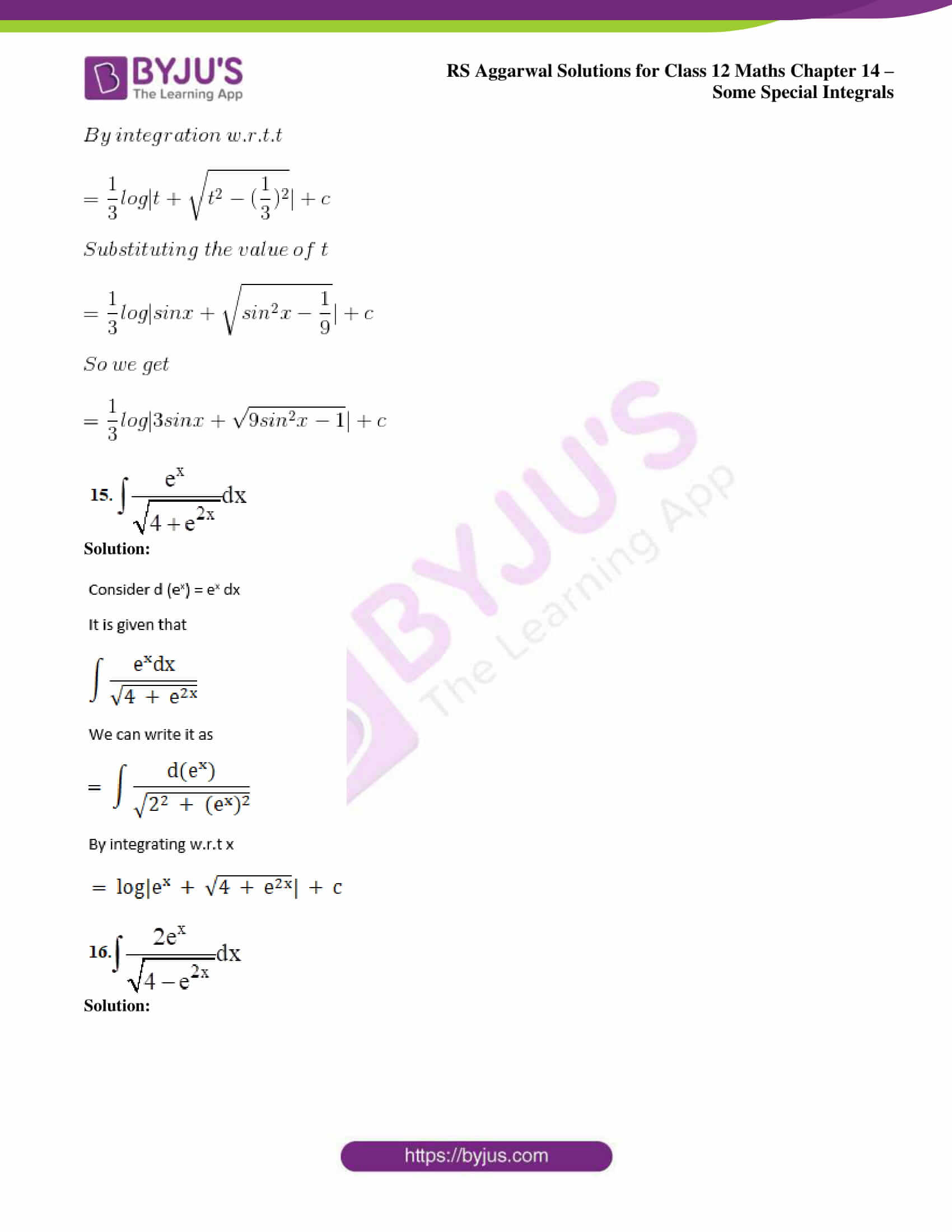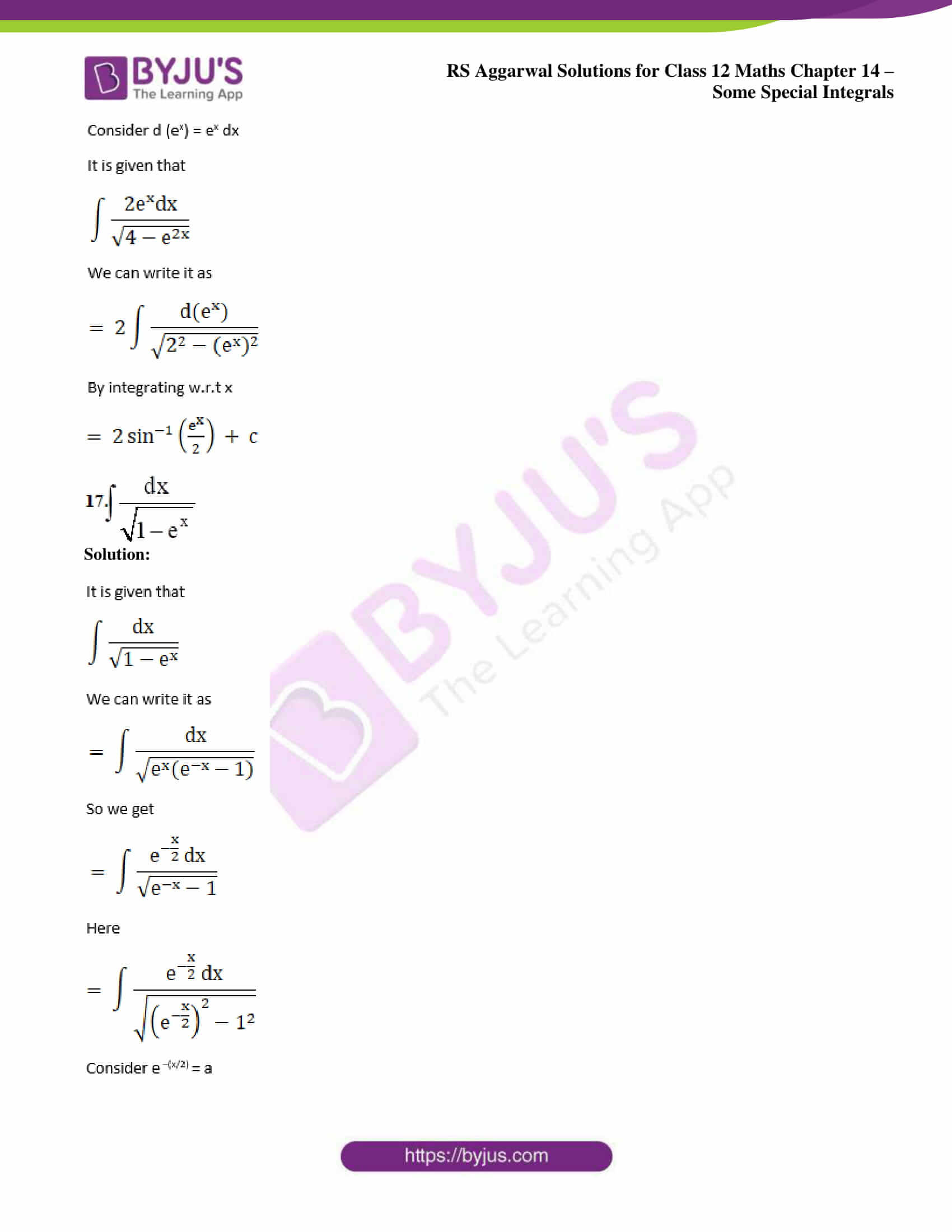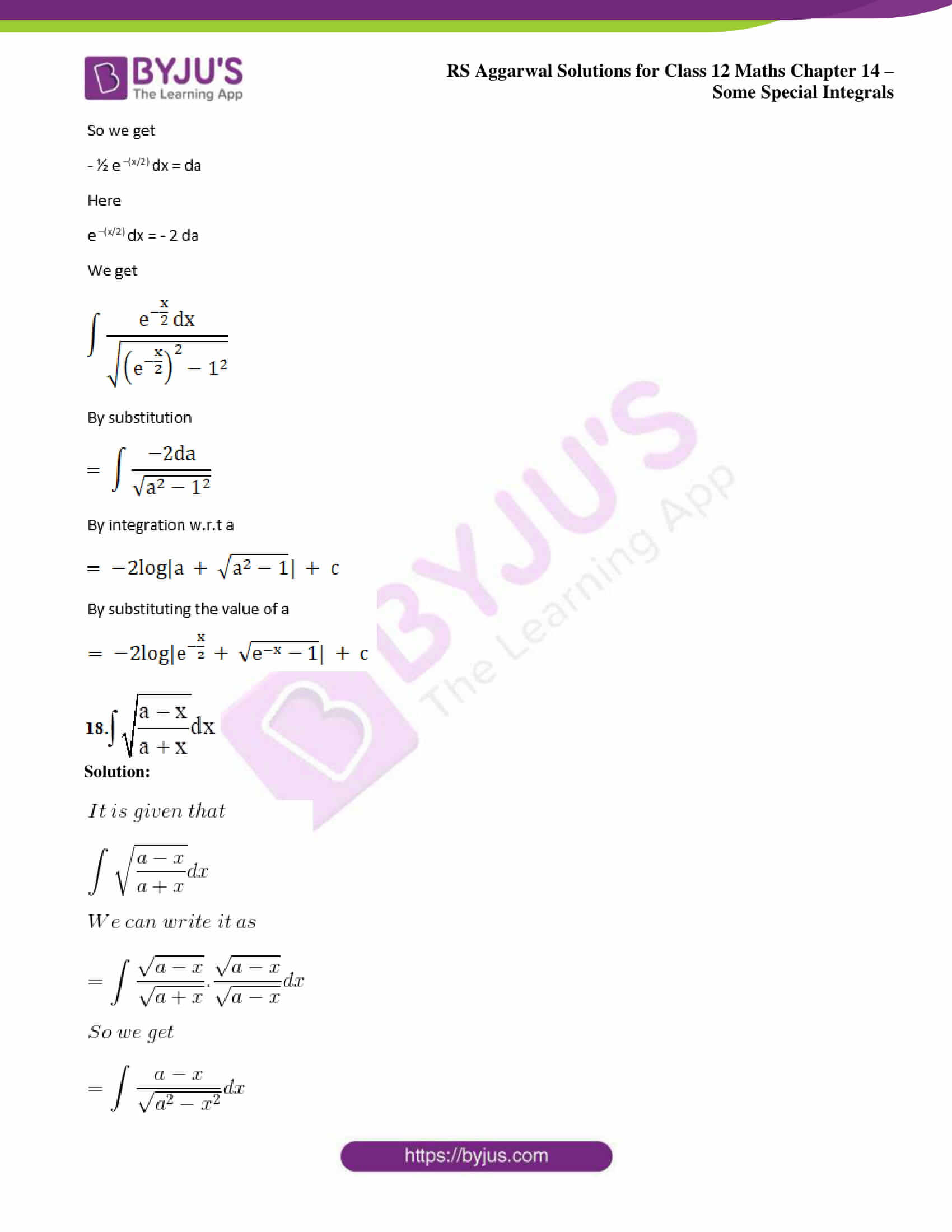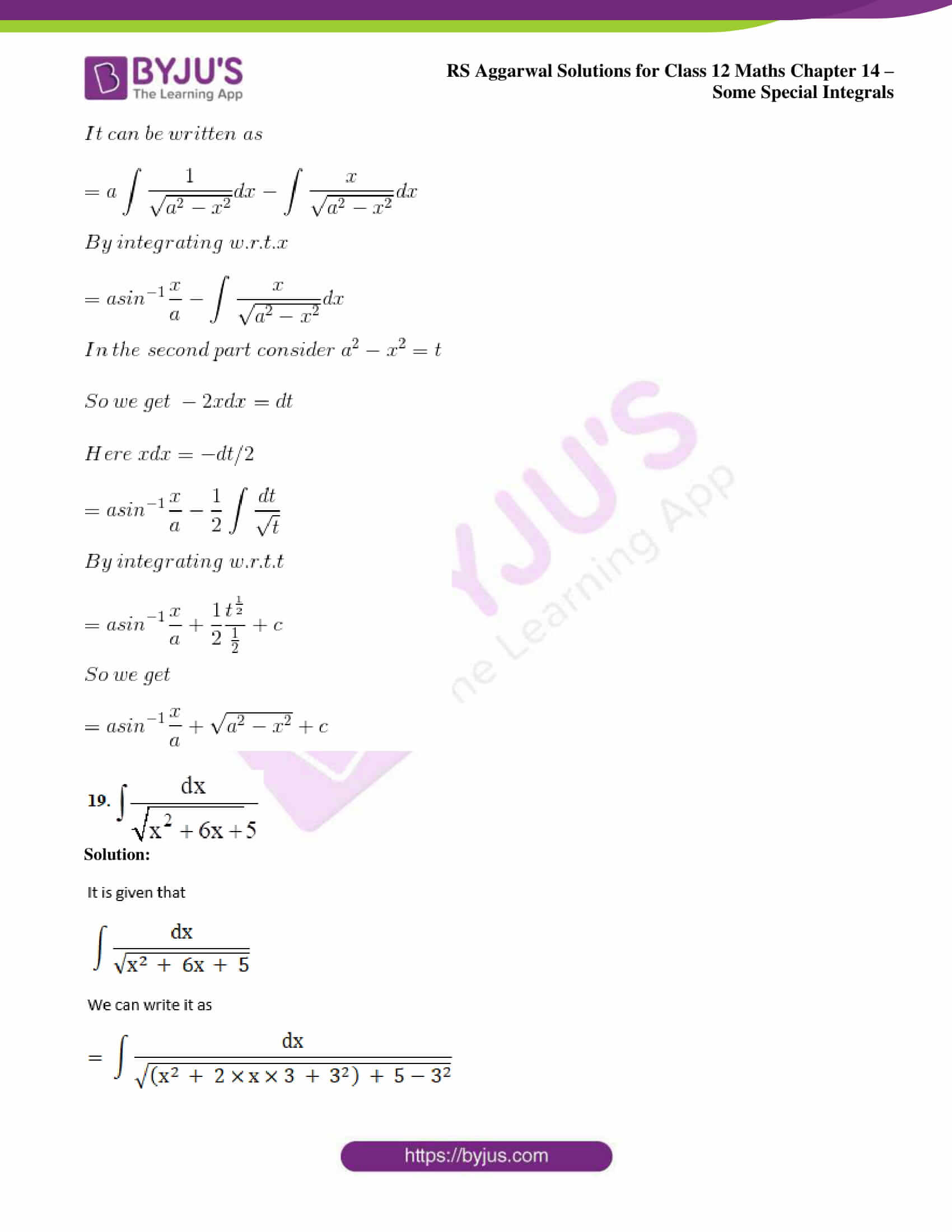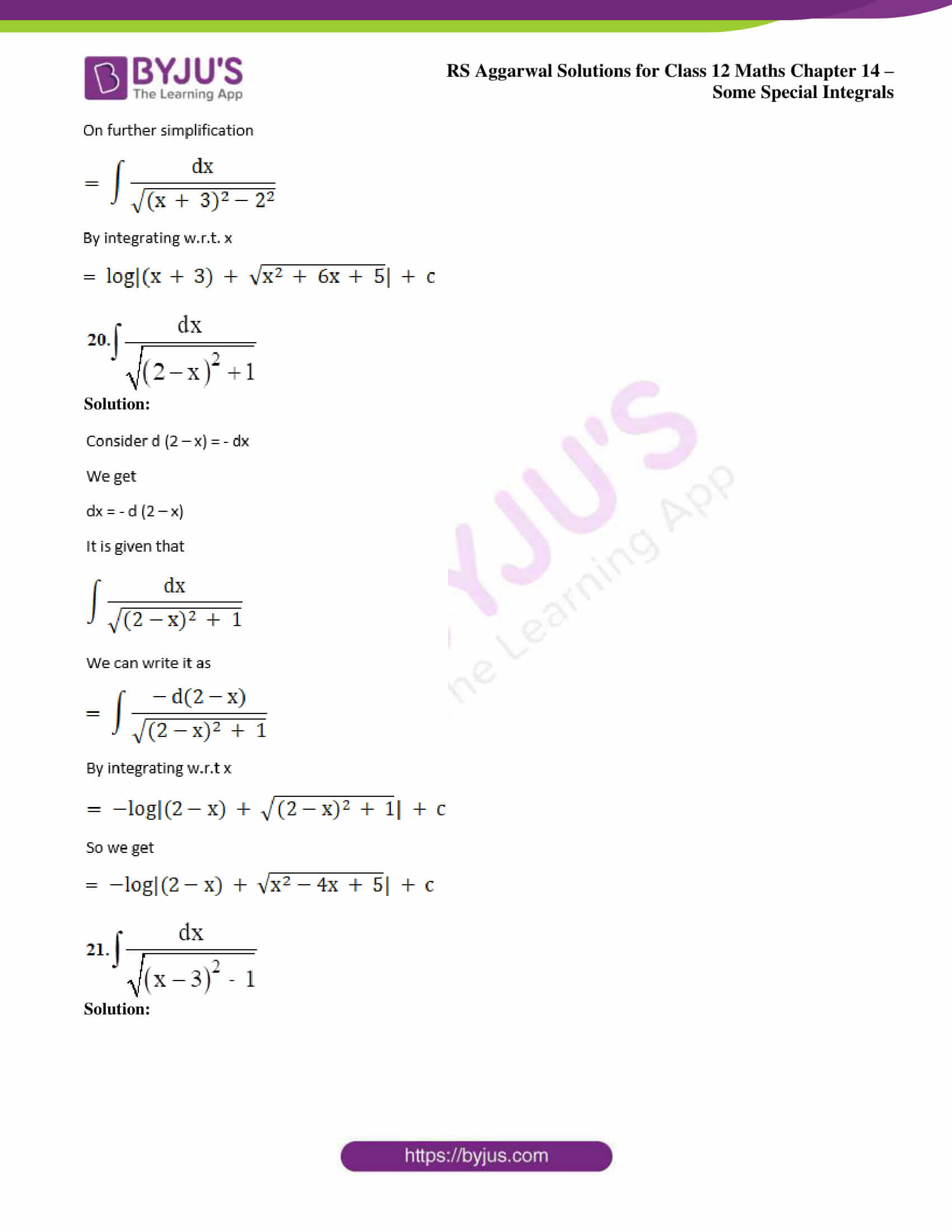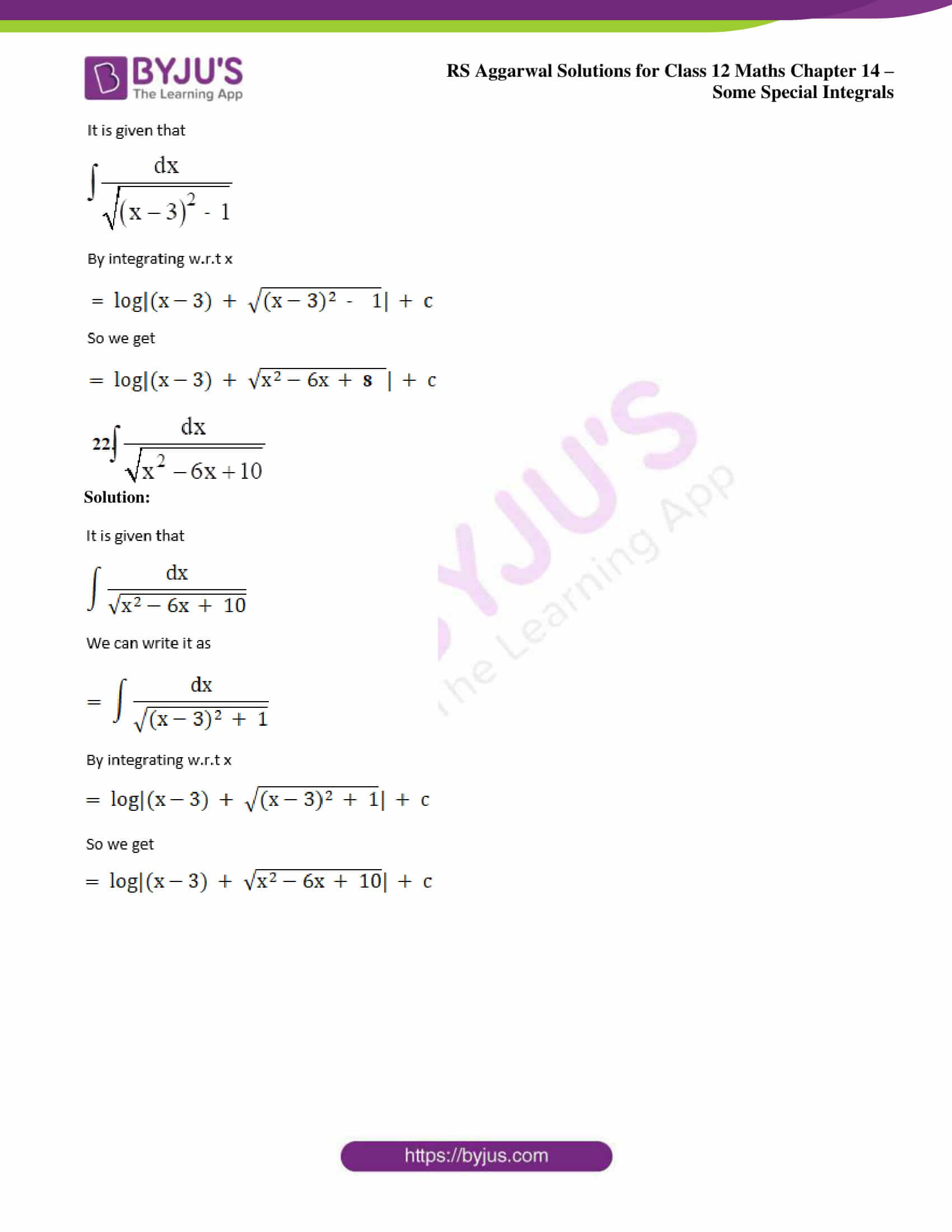### Access other exercise solutions of Class 12 Maths Chapter 14: Some Special Integrals

Exercise 14A Solutions

Exercise 14C Solutions

### Access RS Aggarwal Solutions for Class 12 Maths Chapter 14: Some Special Integrals Exercise 14B

Evaluate: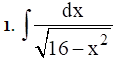Solution: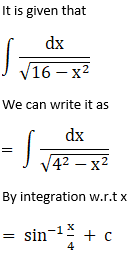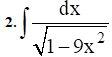Solution: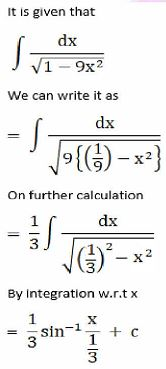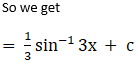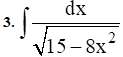Solution: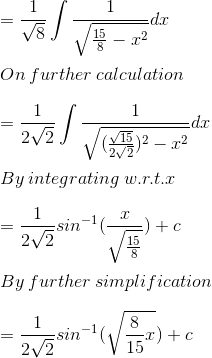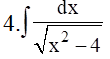Solution: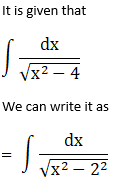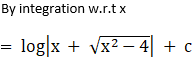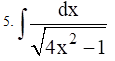Solution: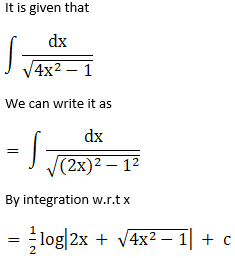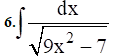Solution: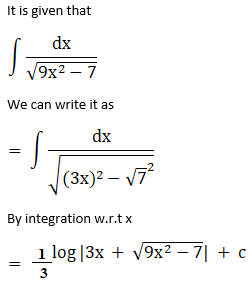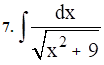Solution: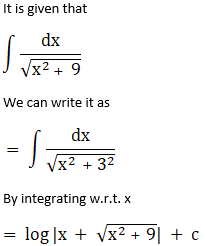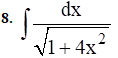Solution: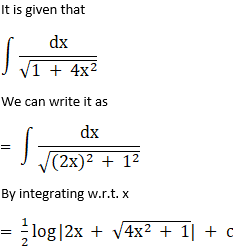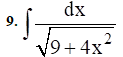Solution: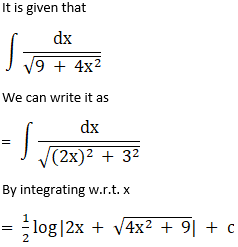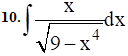Solution: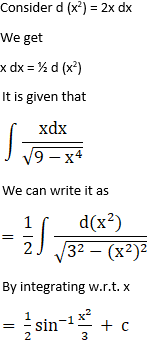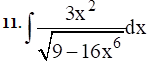Solution: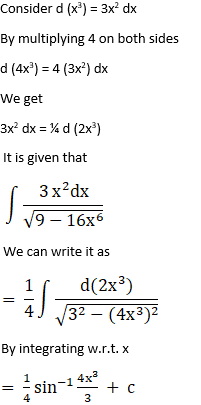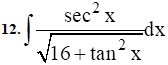Solution: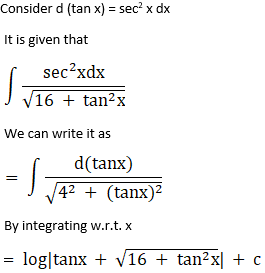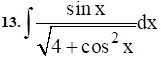Solution: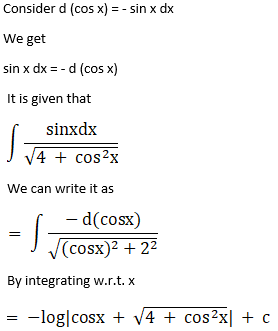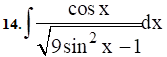Solution: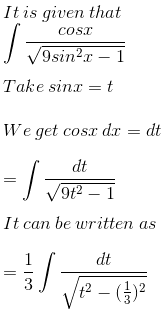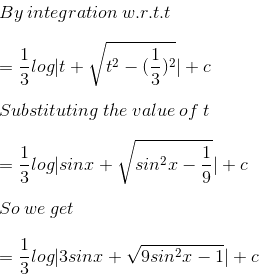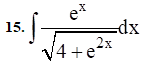Solution: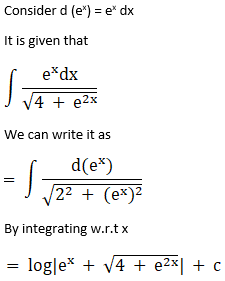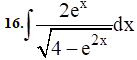Solution: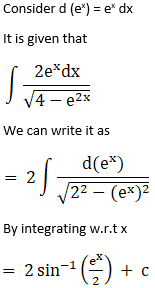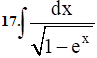Solution: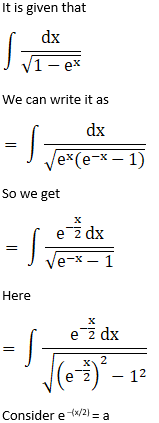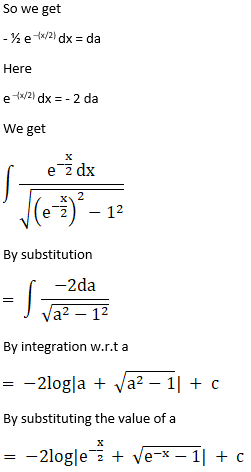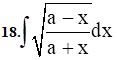Solution: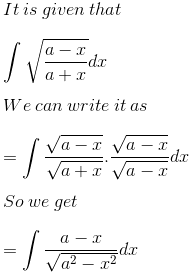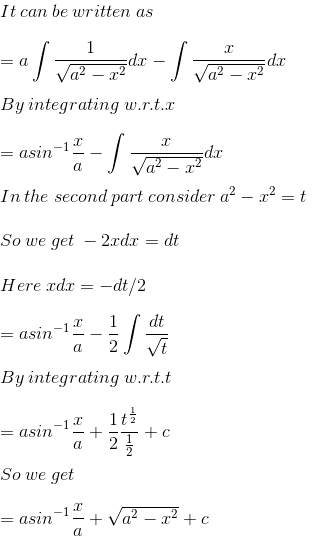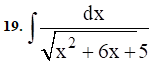Solution: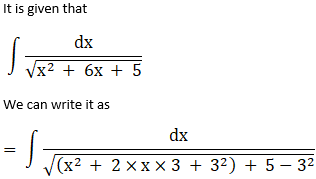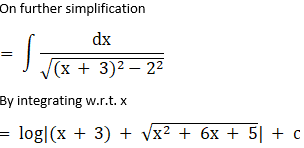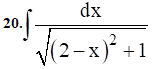Solution: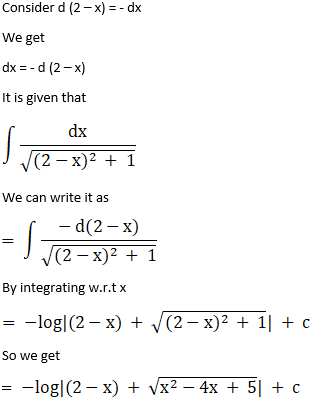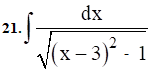Solution: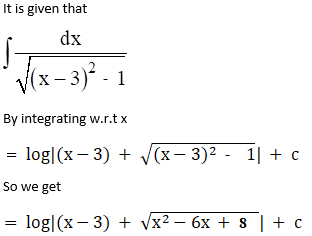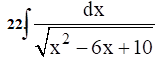Solution: